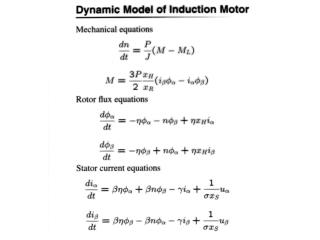DownloadDownload PresentationDC/AC Converter Control Torque and Flux Control

# DC/AC Converter Control Torque and Flux Control

Télécharger la présentation## DC/AC Converter Control Torque and Flux Control

- - - - - - - - - - - - - - - - - - - - - - - - - - - E N D - - - - - - - - - - - - - - - - - - - - - - - - - - -
##### Presentation Transcript

1. DC/AC Converter ControlTorque and Flux Control • Converter circuit + Motor • Control design • Design sliding surface for torque and flux • Lyapunov function denote

2. Torque and Flux Control (cont.) • Calculate where does not depend on control and

3. Torque and Flux Control (cont.) • Select control logic such that Control Logic tends to zero

4. Torque & Flux Control (approach 2) • Cascade Control • From torque and flux equations: where • Desired can be calculated • Sliding mode to provide desired current

5. Cascade Sliding Mode Control • Idea: Utilize extra degree of freedom • Sliding surface design

6. Lyapunov Approach • Select control logic based on Lyapunov function such that sliding mode is enforced • Advantage: simple Control Logic

7. Decoupling Approach to Enforce Sliding Mode • Idea: to decouple motions in • Method: non-singular transformation: • Advantage: allows frequency analysis performed for each surface individually Control Logic

8. Vn Control • Objective: optimality by changing • Example: switching frequency reduction

9. SMPWM Simulation Results • Current tracking and vn tracking

10. Experimental Setup • Controller: TMS320F2812 DSP • Switching devices: 2MBI100NC-12 IGBT • 3 phase RL load • 50 V DC supply

11. Experimental Results The first waveforms show the current tracking (and ), the second ones show tracking as a sinusoidal function by the average value of (after filtering out a high frequency component of discontinuous function ).

12. Neutral Point Voltage Control